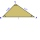# Triangle ABC v2

Area of the triangle is 12 cm square. Angle ACB = 30º , AC = (x + 2) cm, BC = x cm. Calculate the value of x.

Result

x =  -8 cm

#### Solution:Checkout calculation with our calculator of quadratic equations.

Try calculation via our triangle calculator.

Leave us a comment of example and its solution (i.e. if it is still somewhat unclear...):Be the first to comment!#### To solve this verbal math problem are needed these knowledge from mathematics:

Looking for help with calculating roots of a quadratic equation? Do you have a linear equation or system of equations and looking for its solution? Or do you have quadratic equation? See also our right triangle calculator. See also our trigonometric triangle calculator.

## Next similar examples:

1. Triangle SASCalculate the area and perimeter of the triangle, if the two sides are 51 cm and 110 cm long and angle them clamped is 130 °.
2. SquarePoints A[-5,-6] and B[7,-1] are adjacent vertices of the square ABCD. Calculate the area of the square ABCD.
3. RhombusCalculate the perimeter and area of ​​a rhombus whose diagonals are 38 cm and 55 cm long.
4. Area of RTCalculate the area of a right triangle which hypotenuse has length 10 and one hypotenuse segment has lenght 5.
5. GardenArea of a square garden is 6/4 of triangle garden with sides 56 m, 35 m, and 35 m. How many meters of fencing need to fence a square garden?
6. Tetrahedral pyramidWhat is the surface of a regular tetrahedral (four-sided) pyramid if the base edge a=7 and height v=6?
7. Trapezoid MOThe rectangular trapezoid ABCD with right angle at point B, |AC| = 12, |CD| = 8, diagonals are perpendicular to each other. Calculate the perimeter and area of ​​the trapezoid.
8. Three altitudesA triangle with altitudes 4; 5 and 6 cm is given. Calculate the lengths of all medians and all sides in a triangle.
9. Right ΔA right triangle has the length of one leg 28 cm and length of the hypotenuse 53 cm. Calculate the height of the triangle.
10. Regular 5-gonCalculate area of the regular pentagon with side 7 cm.
11. Isosceles triangle 9Given an isosceles triangle ABC where AB= AC. The perimeter is 64cm and altitude is 24cm. Find the area of the isosceles triangle
12. Right triangle AlefThe obvod of a right triangle is 84 cm, the hypotenuse is 37 cm long. Determine the lengths of the legs.
13. RectangleIn rectangle with sides, 6 and 3 mark the diagonal. What is the probability that a randomly selected point within the rectangle is closer to the diagonal than to any side of the rectangle?
14. TerezaThe cube has area of base 256 mm2. Calculate the edge length, volume and area of its surface.
15. CirclesThe areas of the two circles are in the ratio 2:20. The larger circle has diameter 20. Calculate the radius of the smaller circle.
16. Water depthHow many square meters of the inside of the pool wet the water if the pool is 25 meters long and 10 meters wide and the water depth is 1.2 meters everywhere.
17. ScaleSwimming pool is long 110 m and 30 m wide. The plan of the city is shown as a rectangle with area 8.25 cm2. What scale is the city plan?